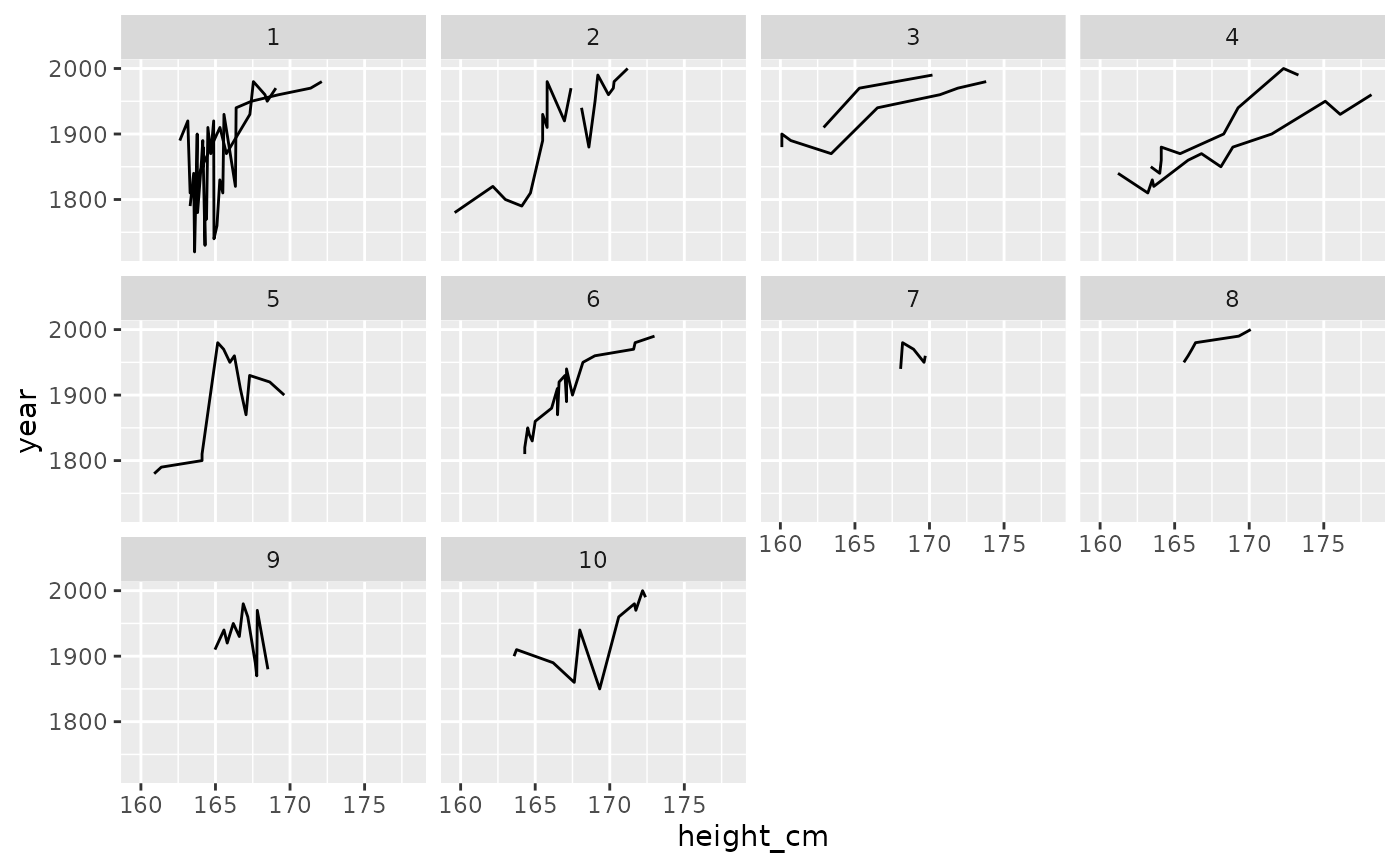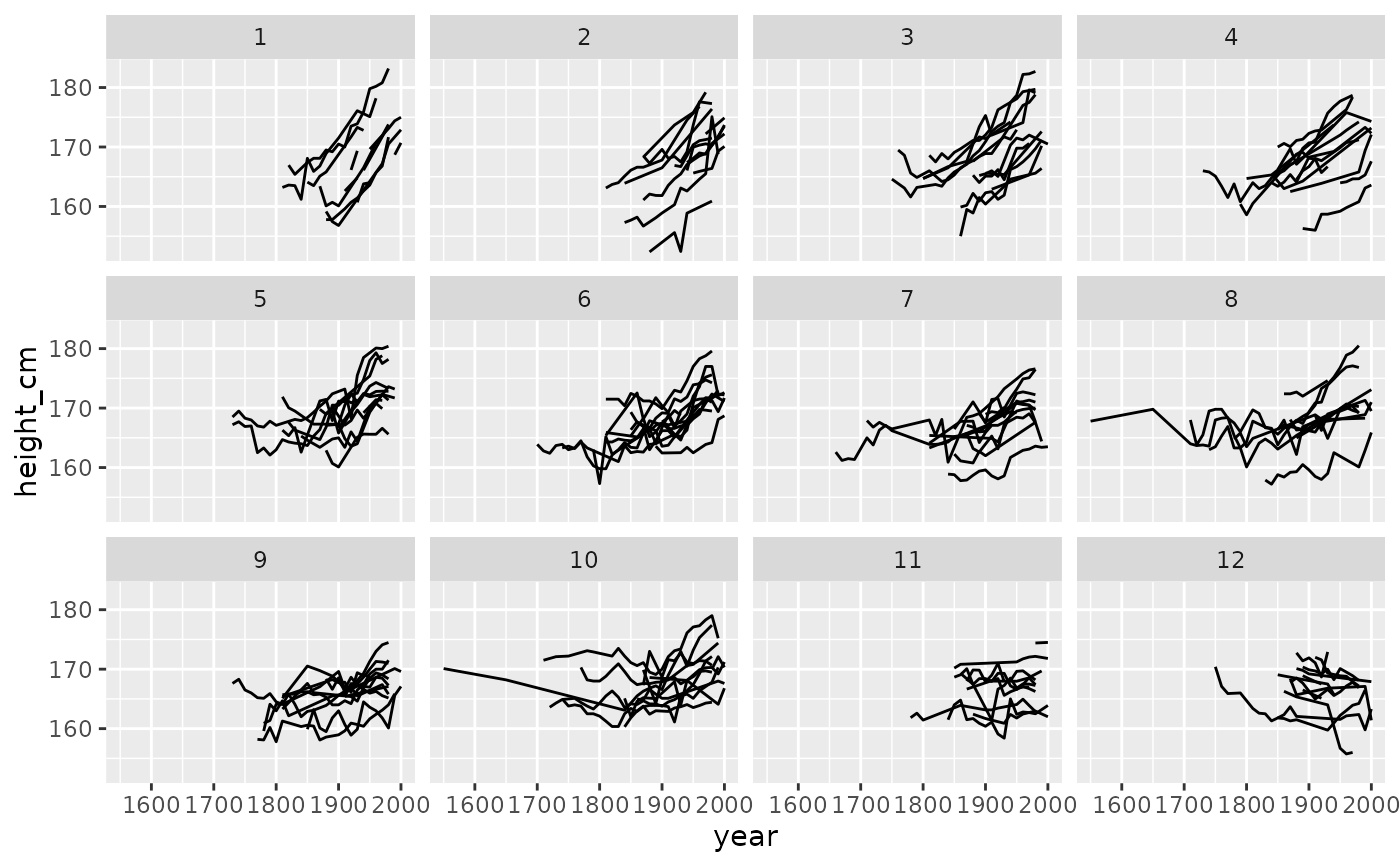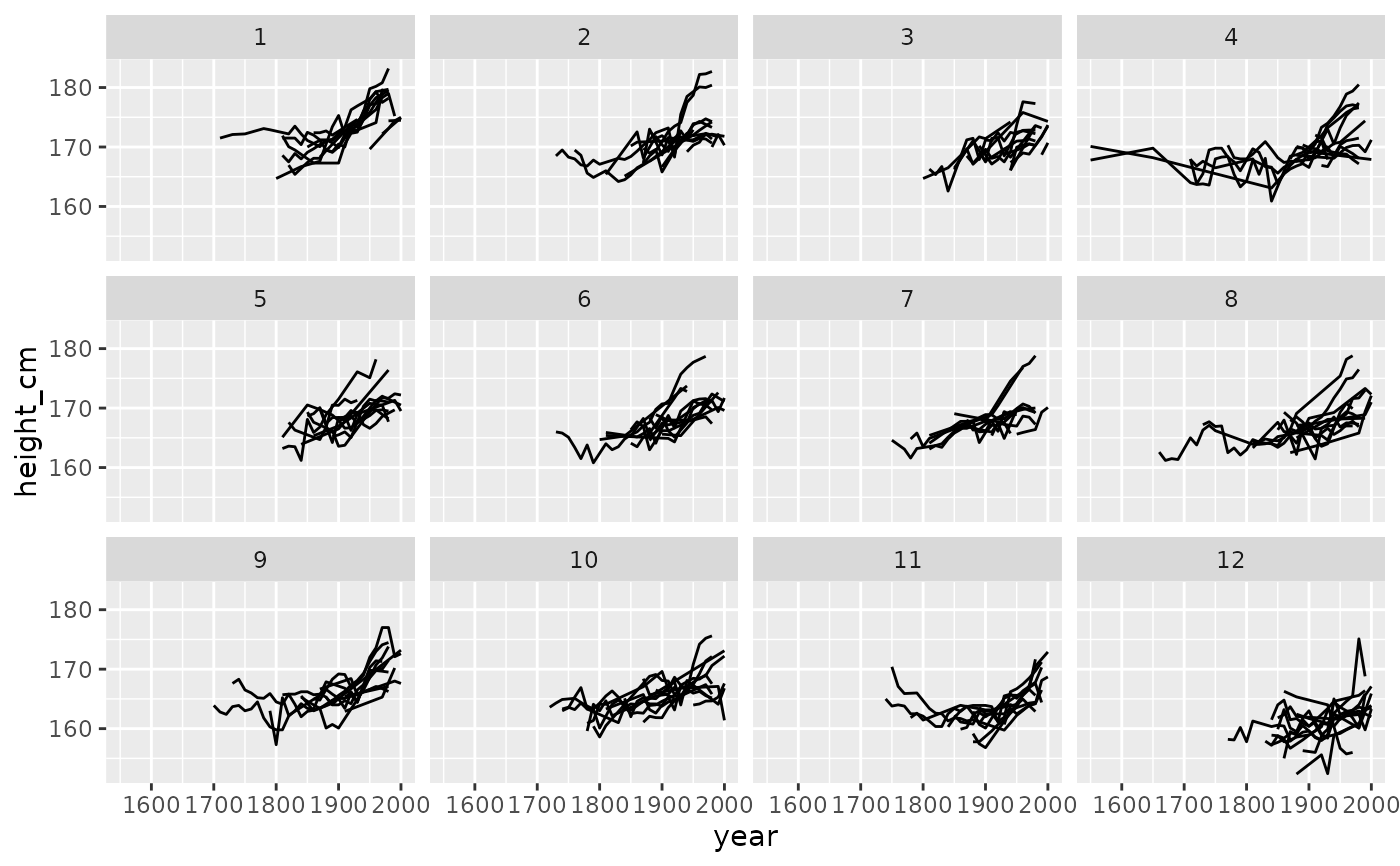To look at as much of the raw data as possible, it can be helpful to stratify the data into groups for plotting. You can stratify the keys using the stratify_keys() function, which adds the column, .strata. This allows the user to create facetted plots showing a more of the raw data.

## Usage

stratify_keys(.data, n_strata, along = NULL, fun = mean, ...)

## Arguments

.data

data.frame to explore

n_strata

number of groups to create

along

variable to stratify along. This groups by each key and then takes a summary statistic (by default, the mean). It then arranges by the mean value for each key and assigns the n_strata groups.

fun

summary function. Default is mean.

...

extra arguments

## Value

data.frame with column, .strata containing n_strata groups

## Examples

library(ggplot2)
library(brolgar)

heights %>%
sample_frac_keys(size = 0.1) %>%
stratify_keys(10) %>%
ggplot(aes(x = height_cm,
y = year,
group = country)) +
geom_line() +
facet_wrap(~.strata)# now facet along some feature
library(dplyr)
heights %>%
key_slope(height_cm ~ year) %>%
right_join(heights, ., by = "country") %>%
stratify_keys(n_strata = 12,
along = .slope_year,
fun = median) %>%
ggplot(aes(x = year,
y = height_cm,
group = country)) +
geom_line() +
facet_wrap(~.strata)heights %>%
stratify_keys(n_strata = 12,
along = height_cm) %>%
ggplot(aes(x = year,
y = height_cm,
group = country)) +
geom_line() +
facet_wrap(~.strata)